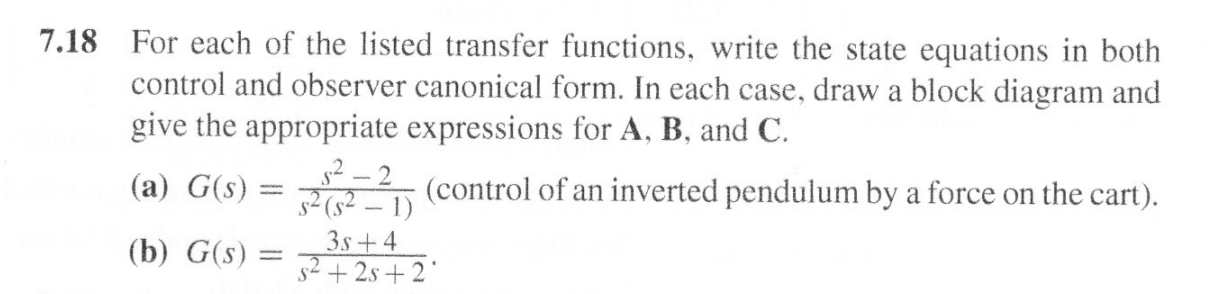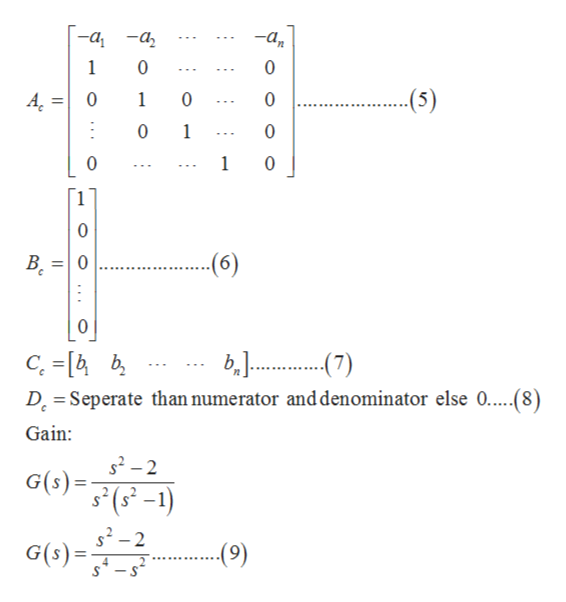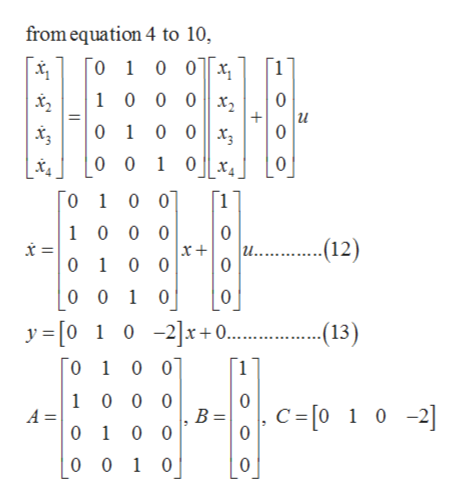Question

# Feedback Control of Dynamic Systems (7th edition) - Problem 7.18help_outlineImage TranscriptioncloseFor each of the listed transfer functions, write the state equations in both control and observer canonical form. In each case, draw a block diagram and give the appropriate expressions for A, B, and C 7.18 2(control of an inverted pendulum by a force on the cart). 2(2-1) (a) G(s) 3s+4 (b) G(s) s2+2s+2 fullscreen
check_circle

Step 1

(a). Numerator part of the gain:

b(s) = b1sn-1 + b2sn-2 + …………+ bn…………………… (1)

Denominator part of the gain:

a(s) = a1sn-1 + a2sn-2 + …………+ an…………………… (2)

General equation:

G(s) = b(s) / a(s)……………… (3)

G(s) = (b1sn-1 + b2sn-2 + …………+ bn) / (a1sn-1 + a2sn-2 + …………+ an) ………………… (4)

Matrices of control canonical form are:help_outlineImage Transcriptionclosea a 1 0 --(5) A 0 1 0 0 10 1 0 0 0 (6) 0 C. -[b D Seperate than numerator and denominator else 0.....(8) Gain 2-2 s2-2 G(s)= ...e) fullscreen
Step 2

General state space equation and output equation are given by:

dx/dt = Ax + Bu ……………. (10)

y = Cx + D………………. (11)

Equations in control canonical form will be:help_outlineImage Transcriptionclosefrom equation 4 to 10, [o 1 0 0x 1 0 0 0 x2 1 0 0 10 0 x 0 0 0 1 0x4 0 1 0 0 10 0 0 0 u.. 0 12) 0 1 0 0 0 0 10 0 .(13) y [0 10 -2]x+0... [0 1 0 0 1 0 0 0 A = 0 1 0 0 0 B = Kc=[o 1 0 -2] 0 0 1 0 fullscreen
Step 3

Block diagram can be formed using equa...

### Want to see the full answer?

See Solution

#### Want to see this answer and more?

Solutions are written by subject experts who are available 24/7. Questions are typically answered within 1 hour.*

See Solution
*Response times may vary by subject and question.
Tagged in

### Electrical Engineering